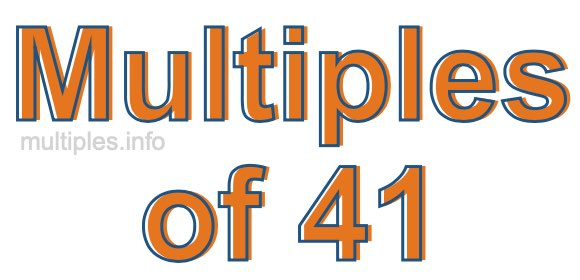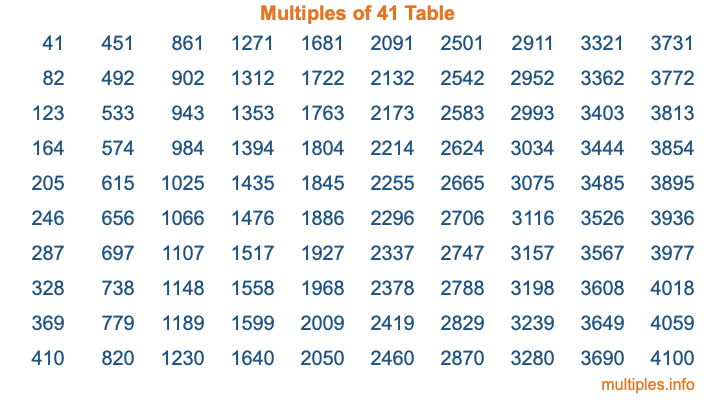Multiples of 41Welcome to the Multiples of 41 page. Here we will first teach you everything you will ever need to know about the multiples of 41, and then give you a study guide summary of everything we taught you to make sure you remember it all. Use this page to look up facts and learn information about the multiples of 41. This page will make you a multiples of forty-one expert!

Definition of Multiples of 41
Multiples of 41 are all the numbers that when divided by 41 equal an integer. Each of the multiples of 41 are called a multiple. A multiple of 41 is created by multiplying 41 by an integer.

Therefore, to create a list of multiples of 41, you start with 1 multiplied by 41, then 2 multiplied by 41, then 3 multiplied by 41, and so on for as long as you want. Thus, the list of the first five multiples of 41 is 41, 82, 123, 164, and 205. To see a larger list of multiples of 41, see the printable image of Multiples of 41 further down on this page. We also have a category where you can choose any nth multiple of 41.

Multiples of 41 Checker
The Multiples of 41 Checker below checks to see if any number of your choice is a multiple of 41. In other words, it checks to see if there is any number (integer) that when multiplied by 41 will equal your number. To do that, we divide your number by 41. If the the quotient is an integer, then your number is a multiple of 41.

Is  a multiple of 41?

Least Common Multiple of 41 and ...
A Least Common Multiple (LCM) is the lowest multiple that two or more numbers have in common. This is also called the smallest common multiple or lowest common multiple and is useful to know when you are adding our subtracting fractions. Enter one or more numbers below (41 is already entered) to find the LCM.

Check out our LCM Calculator if you need more details about the Least Common Multiple or if you need the LCM for different numbers for adding and subtraction fractions.

nth Multiple of 41
As we stated above, 41 is the first multiple of 41, 82 is the second multiple of 41, 123 is the third multiple of 41, and so on. Enter a number below to find the nth multiple of 41.

th multiple of 41

Multiples of 41 vs Factors of 41
41 is a multiple of 41 and a factor of 41, but that is where the similarities end. All postive multiples of 41 are 41 or greater than 41. All positive factors of 41 are 41 or less than 41.

Below is the beginning list of multiples of 41 and the factors of 41 so you can compare:

Multiples of 41: 41, 82, 123, 164, 205, etc.

Factors of 41: 1, 41

As you can see, the multiples of 41 are all the numbers that you can divide by 41 to get a whole number. The factors of 41, on the other hand, are all the whole numbers that you can multiply by another whole number to get 41.

It's also interesting to note that if a number (x) is a factor of 41, then 41 will also be a multiple of that number (x).

Multiples of 41 vs Divisors of 41
The divisors of 41 are all the integers that 41 can be divided by evenly. Below is a list of the divisors of 41.

Divisors of 41: 1, 41

The interesting thing to note here is that if you take any multiple of 41 and divide it by a divisor of 41, you will see that the quotient is an integer.

Multiples of 41 Table
Below is an image of the first 100 multiples of 41 in a table. The table is in chronological order, column by column. The first column has the first ten multiples of 41, the second column has the next ten multiples of 41, and so on.The Multiples of 41 Table is also referred to as the 41 Times Table or Times Table of 41. You are welcome to print out our table for your studies.

Negative Multiples of 41
Although not often discussed or needed in math, it is worth mentioning that you can make a list of negative multiples of 41 by multiplying 41 by -1, then by -2, then by -3, and so on, to get the following list of negative multiples of 41:

-41, -82, -123, -164, -205, etc.

Multiples of 41 Summary
Below is a summary of important Multiples of 41 facts that we have discussed on this page. To retain the knowledge on this page, we recommend that you read through the summary and explain to yourself or a study partner why they hold true.

There are an infinite number of multiples of 41.

A multiple of 41 divided by 41 will equal a whole number.

41 divided by a factor of 41 equals a divisor of 41.

The nth multiple of 41 is n times 41.

The largest factor of 41 is equal to the first positive multiple of 41.

41 is a multiple of every factor of 41.

41 is a multiple of 41.

A multiple of 41 divided by a divisor of 41 equals an integer.

41 divided by a divisor of 41 equals a factor of 41.

Any integer times 41 will equal a multiple of 41.

Multiples of a Number
Here you can get the multiples of another number, all with the same attention to detail as we did for multiples of 41 on this page.

Multiples of
Multiples of 42
Did you find our page about multiples of forty-one educational? Do you want more knowledge? Check out the multiples of the next number on our list!